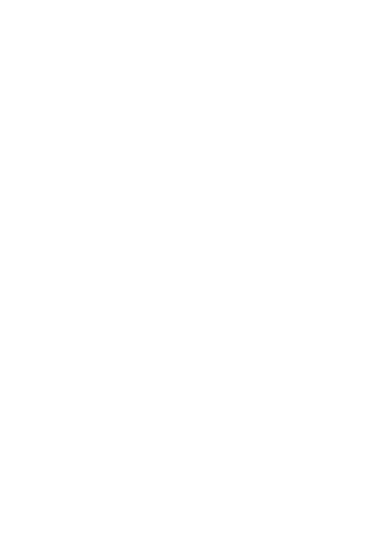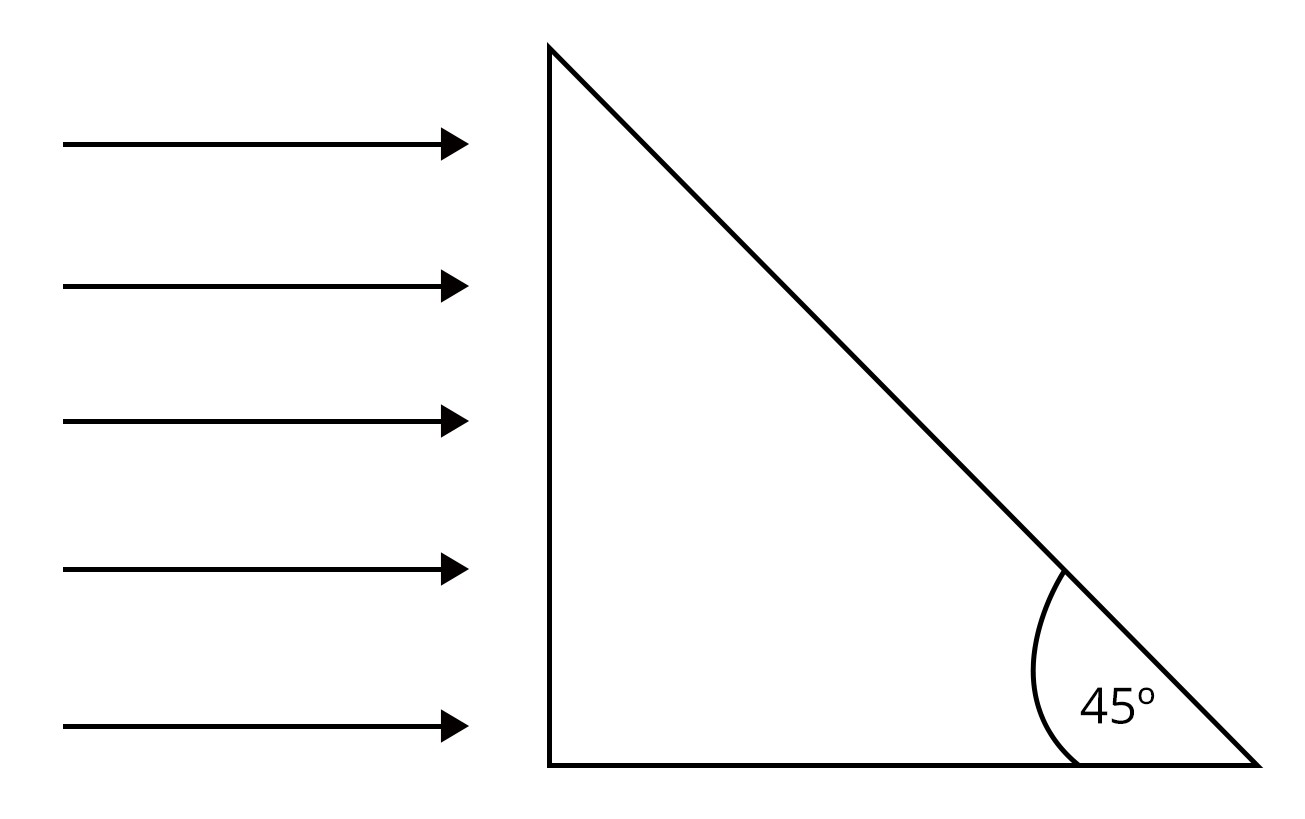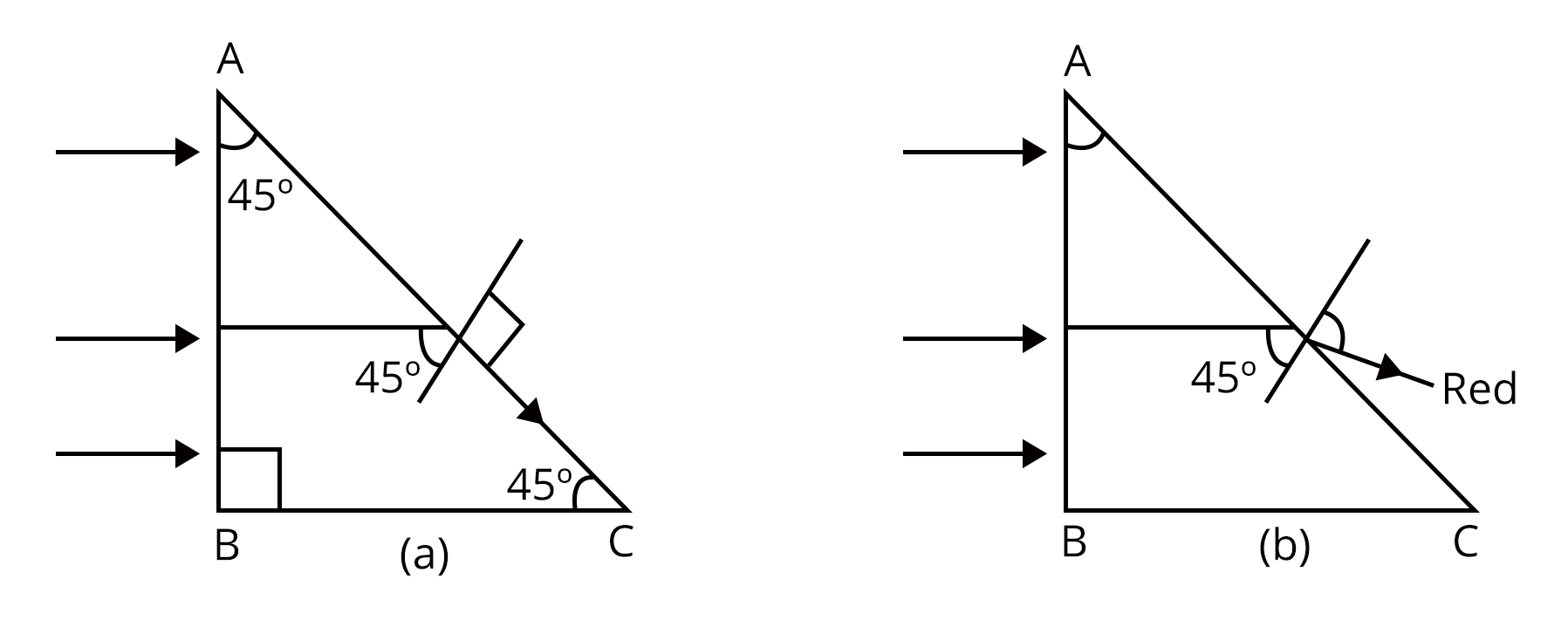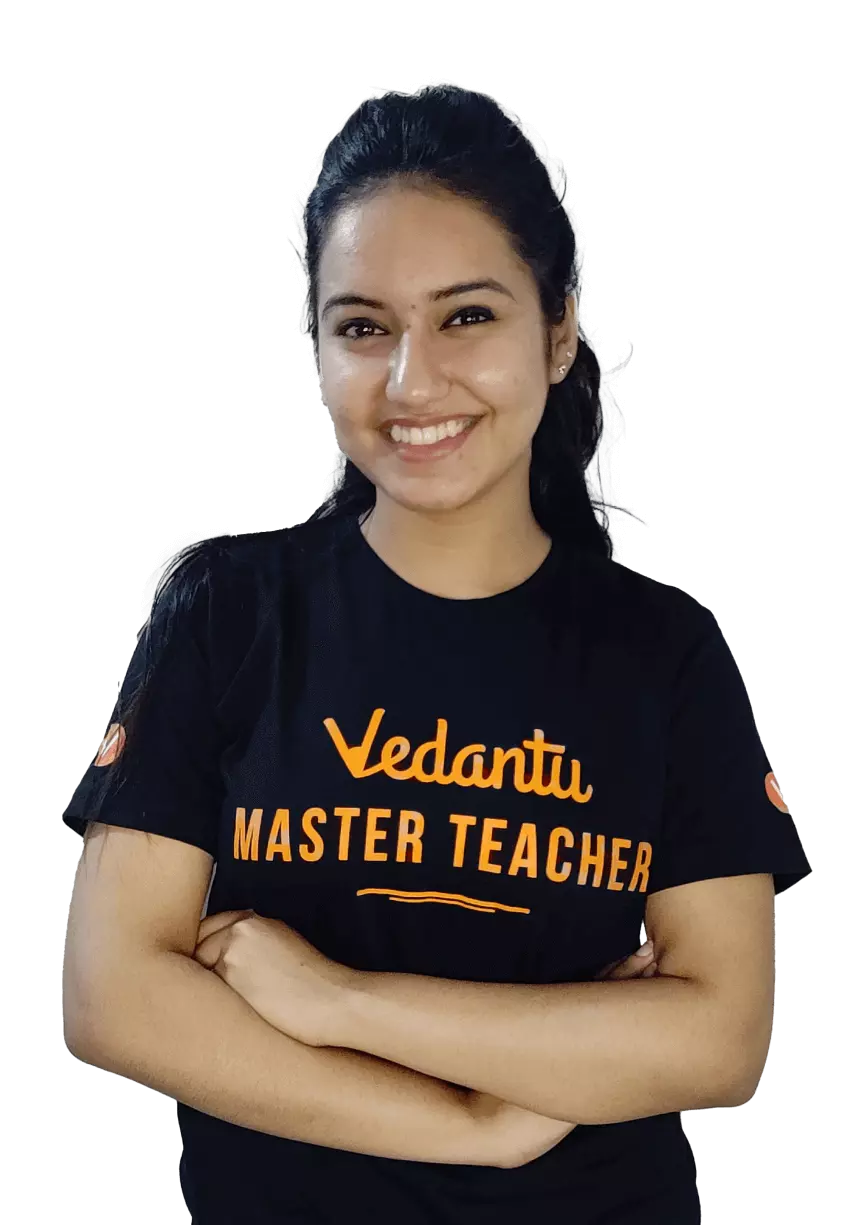JEE## Important Concept of Optics for JEE

This chapter gives us the answer to a few interesting questions like “what is optics”, “what is light” and “what are its various properties and applications”. Optics is one of the branches of physics in which we study the behaviour of light and how under different circumstances, its properties get altered. The chapter also includes various examples of optics, types of optics, theory of optics and uses of optics in our day to day life.

The major concepts of this chapter include:

• Reflection of Light: This topic includes the behaviour of light when it falls on the surface of plane mirror and curved mirror and also gives the laws related to it.

• Refraction of Light: This topic tells us about how a ray of light travels when it is passed through a rare medium to a denser medium and vice-versa.

• Dispersion of Light: This topic deals with what happens to a ray of light when it passes through a prism.

• Optical Instruments: This topic includes various types of optical instruments like simple microscope, compound microscope, astronomical telescope and reflecting type telescope.

• Huygens Principle and Interference of Light: In this topic, we study the wave nature of light and what happens when two waves are superimposed on each other.

• Diffraction and Polarisation: This topic gives us answers about what happens to a ray of light when it passes through a single slit and how we can restrict the electric vector of the light.

Now, let's move on to the important concepts and formulae related to JEE and JEE Main exams along with a few solved examples.

### Important Topics of Optics

• Reflection of light

• Refraction of light

• Dispersion of light

• Optical instruments

• Huygens principle and interference of light

• Diffraction and polarisation of light

### Optics Important Concepts for JEE

 Name of the Concept Key Points of the Concepts 1. Reflection of light The phenomenon of light reflection is the change in the course of light without any change in the medium.The mirror formula is a relationship between the mirror's focal length and the distance between the object and image from the mirror.Magnification is defined as the ratio of the size of the image formed by the mirror to the size of the object. 2. Refraction of light Refraction of light is the phenomenon of change in the path of light when it goes from one medium to another.The basic cause of refraction is change in the velocity of light when it goes from one medium to the other.The phenomenon of total internal reflection is the reflection of light into a denser medium from an interface of this denser medium and a rarer medium.Spherical reflecting surfaces is a part of a sphere of transparent medium and these are of two types:Convex spherical refracting surface, which is convex towards the rarer medium side. Concave  spherical refracting surface, which is concave towards the rarer medium side.A lens is a section of a transparent refracting material that is joined by two spherical surfaces or one spherical surface and one plane surface. There are types of lenses:Converging or Convex lensDiverging or Concave lensLens maker’s formula is a relation that connects focal length($f$) of the lens to radii of curvature ($R_1$ and $R_2$) of the two surfaces of the lens and refractive index($\mu$) of the material of the lens. It is written as$\frac{1}{f}=(\mu-1)(\frac{1}{R_1}-\frac{1}{R_2})$Power of a lens ($P$) is defined as the ability of the lens to converge a beam of light falling on the lens and it is measured as $P=\dfrac{1}{f}$ 3. Dispersion of light The phenomenon of light dispersion is the splitting of a white light beam into its constituent colours when it passes through a prism.The ratio of angular dispersion to the mean deviation generated by a prism is known as its dispersive power.The dispersive power of a prism is solely determined by the type of prism material. However, angular dispersion and mean deviation, both depend on angle of prism in addition to the nature of material of the prism. 4. Optical instruments A simple microscope is used for observing magnified images of tiny objects and it consists of a converging lens of small focal length.Magnifying power ($m$) of a simple microscope is defined as the ratio of angles subtended by the image and the object on the eye, when both are at the least distance of distinct vision($d$) from the eye. It is given as$m=(1+\frac{d}{f})$Compound microscope consists of two lenses co-axially at the free end of a tube, at a suitable fixed distance from each other.The magnifying power of the compound microscope is given as$m=\frac{L}{|f_o|}(1+\frac{d}{f_e})$Here, $f_o$= focal length of objective lens, $f_e$= focal length of the eye-piece, $L$= length of the microscope tube and $d$= least distance of distinct vision.Astronomical telescope consists of two lenses i.e, an objective lens which is of large focal length($f_o$) and an eyepiece which has a small focal length($f_e$).The astronomical telescope's magnification power is given as$m=\frac{f_o}{-f_e}$Reflecting type of telescope consists of a parabolic concave reflector with a narrow hole at the centre and secondary convex mirror.In normal adjustment, magnifying power of the a reflecting type telescope is given as$m=\frac{R/2}{f_e}$Here, $R$= radius of curvature of concave reflector and $f_e$ = focal length of the eyepiece 5. Huygens principle and interference of light The continuous location of all particles of a material vibrating in the same phase is characterised as a wave front.According to Huygen’s principle,Every particle on the given wavefront acts as a fresh source of new disturbance, called secondary wavelets, which travels in all directions with the velocity of light in the medium.At any instant, a surface encountering these secondary wavelets tangentially in the forward direction yields the new wavefront at that instant. This is referred to as a secondary wave front.The process of redistribution of light energy in a medium caused by the superposition of light waves from two coherent sources is known as interference of light.Condition for constructive interference:$x=n\lambda$Here, $x$= path difference between two waves, $\lambda$= wavelength of light and $n$= 0,1,2……..Condition for destructive interference:$x=\frac{(2n-1)\lambda}{2}$Here, $n$ = 1,2,3………….In Young’s double slit experiment,The condition for bright fringe is given as$x=n\lambda(\frac{D}{d})$Here, $n$ = 0,1,2,3………The condition for dark fringe is$x=(2n-1)\frac{\lambda}{2}\frac{D}{d}$Here, $D$= distance between slit and screen, $d$ = distance between two slits and $n$ = 1,2,3……….The expression of fringe width ($\beta$) is$\beta=\frac{\lambda D}{d}$ 6. Diffraction and polarisation of light The phenomenon of light bending around corners of a barrier or aperture in its path is known as diffraction.The $n^{th}$ secondary minima is given as$\sin\theta_n=\frac{n\lambda}{a}$The $n^{th}$ secondary maxima is given as$\sin\theta_n=\frac{(2n+1)\lambda}{2a}$Here, $n$ = 1,2,3……an integer, $a$ = width of the aperture, $\theta$ = angle made by secondary waves while travelling and $\lambda$ = wavelength of light.The phenomenon of restricting the vibration of light in a particular direction, perpendicular to the direction of the wave motion, is called polarisation of light.According to the Law of Malus, when a beam of completely polarised light is incident on an analyser, the resultant intensity of light ($I$) transmitted from the analyser varies directly as the square of the cosine of the angle ($\theta$) between plane of transmission of analyser and polariser.

### List of Important Formulae for Optics

 S.No. Name of the Concept Formula Reflection of light Mirror formula:$\frac{1}{v}+\frac{1}{u}=\frac{1}{f}$Linear magnification of a spherical mirror$m=-\dfrac{v}{u}$ Refraction of light Snell’s Law:$\frac{\mu_2}{\mu_1}=\frac{\sin i}{\sin r}$Here, $\mu_1$ and $\mu_1$ are the refractive indices of the medium 1 and medium 2.Total internal reflection:$\mu^a_w=\frac{1}{\sin C}$Lens maker’s formula:$\frac{1}{f}=(\mu-1)(\frac{1}{R_1}-\frac{1}{R_2})$Magnification for concave lens:$m=\frac{v}{u}$Equivalent focal length ($F$) of two lenses having focal lengths $f_1$, $f_2$ and separated by a distance $d$ is$\frac{1}{F}=\frac{1}{f_1}+\frac{1}{f_2}-\frac{d}{f_1f_2}$ Dispersion of light Refractive index of prism is$\mu=\frac{\sin(A+\delta_m/2)}{\sin (A/2)}$Here, $A$ = angle of prism and $\delta_m$ = minimum deviation angle.Dispersive power ($\omega$) is given as$\omega=\frac{d\mu}{\mu-1}$Here, $d\mu$ = difference in refractive indices of prism for violet and red colour. Optical instruments A simple microscope's magnification power ($m$) equals to$m=(1+\frac{d}{f})$The magnifying power of compound microscope is$m=\frac{L}{|f_o|}(1+\frac{d}{f_e})$Magnifying power of astronomical telescope is$m=\frac{f_o}{-f_e}$Magnifying power of the a reflecting type telescope is$m=\frac{R/2}{f_e}$ Huygens Principle and interference of light In Young’s double slit experiment, the condition for bright fringe is given as$x=n\lambda(\frac{D}{d})$Here, $n$ = 0,1,2,3………The condition for dark fringe is$x=(2n-1)\frac{\lambda}{2}\frac{D}{d}$Here, $n$ = 1,2,3……….Fringe width ($\beta$) is$\beta=\frac{\lambda D}{d}$ 6. Diffraction and polarisation of light The $n^{th}$ secondary minima in diffraction is$\sin\theta_n=\frac{n\lambda}{a}$The $n^{th}$ secondary maxima in diffraction is$\sin\theta_n=\frac{(2n+1)\lambda}{2a}$Law of Malus is$I\varpropto\cos^2\theta$

### Solved Examples

1. When a glass plate with a refractive index of 1.3 is put in the path of one of the beams in Young's double slit experiment, the fringes are displaced by a distance $x$. The fringe shift is $(5/2)x$ when this plate is replaced by another plate of the same thickness. The second plate's refractive index will be_______.

Sol:

Given:

Refractive index of glass plate, $\mu_1 = 1.3$

Shift in the fringes is $(5/2)x$.

To find, $\mu_2 = ?$

To solve this problem, we have to use the relation between the thickness of a plate, path difference and its refractive index. Also, we have to use the relation of fringe width.

The relation between the path difference($\Delta$), the thickness of the plate ($t$) and its refractive index ($\mu$) is given as

$\Delta=(\mu-1)t$

The path difference of $\lambda$ has introduced the phase difference of $\beta$. Therefore, a path difference of $(\mu-1)t$ that will introduce a shift of $x$ is given as

$x=\frac{(\mu-1)t\beta}{\lambda}$

In the case of the first plate, we can write:

$x=\frac{(\mu_1-1)t\beta}{\lambda}$.........(1)

In the case of the second plate, we can write:

$\dfrac{5x}{2}=\frac{(\mu_2-1)t\beta}{\lambda}$.........(2)

After dividing eq. (2) by (1), we get:

$\frac{(\mu_2-1)}{(\mu_1-1)}=\frac{5}{2}$

On further solving, we get:

$\mu_2-1 = \frac{5}{2} (\mu_1-1)$

Now, after putting the value of $\mu_1$, we get:

$\mu_2-1 = \frac{5}{2} (1.3-1)$

$\mu_2-1 =2.5 \times 0.3$

$\mu_2-1 = 0.75$

$\mu_2=0.75+1=1.75$

Therefore, the refractive index of the second plate is 1.75.

Key point: The knowledge of the concept of path difference is essential for solving such problems.

2. The slits in Young's double-slit experiment are spaced by 0.3 mm, and the screen is 130 cm distant. To obtain interference fringes on the screen, a laser beam with two wavelengths, 600 nm and 500 nm, is utilised. Calculate the shortest distance between the shared centre maxima and the point where the bright fringes caused by both wavelengths coincide.

Sol:

Given:

Distance between the slits, $d=0.3\,mm=0.3\times 10^{-3}\,m$

Wavelengths of the laser, $\lambda_1=600\,nm=600 \times 10^{-9}\,m$

And, $\lambda_2=500\,nm=500 \times 10^{-9}\,m$

Distance between slits and screen, $D=130\,cm=1.3\,m$

In order to solve this problem, we have to apply the concept of central maxima in interference pattern. Let $x$ be the distance between the centre maxima and the point at which the bright fringes caused by both wavelengths coincide.

Now for $\lambda_1$, we can write the condition for bright fringe as

$x=\frac{n\lambda_1 D}{d}$......(3)

Similarly, for for $\lambda_2$

$x=\frac{m\lambda_2 D}{d}$........(4)

After dividing the equations (1) and (2), we get:

$\frac{m}{n}=\frac{\lambda_1}{\lambda_2}$

$\frac{m}{n}=\frac{600 \times 10^{-9}}{500 \times 10^{-9}}$

$\frac{m}{n}=\dfrac{6}{5}$

From the above relation, we can conclude that the central maxima of $5^{th}$ bright fringe of $\lambda_1$ coincides with the $6^{th}$ bright fringe of $\lambda_2$.

Now, using equation (3), we can find the value of $x$ as

$x=\frac{n\lambda_1 D}{d}$

$x=\frac{5\times 600 \times 10^{-9} \times 1.3 }{0.3\times 10^{-3}}$

$x= 13000 \times 10^{-6}\,m=0.13\,mm$

Therefore, the shortest distance will be 0.13 mm.

Key point: The concept of interference of light and its conditions for bright fringes is important to solve this problem.

### Previous Years’ Questions from JEE Paper

1. A beam of white light is incident on the glass air interface from glass to air such that green light just suffers total internal reflection. The colours of the light which will come out to air are

1. violet, indigo, blue

2. all colours except green

3. yellow, orange, red

4. white light

Sol:

According to the question, it is given that white light is incident on the glass air interface from glass to air and green light suffers total internal reflection. We know that white light consists of seven bands of colours i.e, VIBGYOR whose wavelength increases as we go from violet to red colour.

Therefore, the wavelengths of yellow, orange and red colour light are greater than the wavelength of green colour light, while the wavelengths of violet, indigo and blue are lesser than green colour light.

The refractive index relation under total internal reflection is given as

$\mu=\frac{1}{\sin C}$

And as $\mu \varpropto \frac{1}{\lambda}$

We can conclude that

$\sin C \varpropto \lambda$

Hence, $C$ will be greater for yellow, orange and red colour light. Therefore, these colour lights will come into the air from the glass.

Key point: The concept of total internal reflection and the relation between refractive index and wavelength of light are the important keys to solve this problem.

2.A beam of light consisting of red, green and blue colours is incident on a right angled prism, as shown in figure. The refractive indices of the material of the prism for the above red, green and blue wavelengths are 1.39, 1.44 and 1.47, respectively. The prism will

a. separate part of the red colour from the green and blue colours.

b. separate part of the blue colour from the red and green colours.

c. separate all the three colours from one another.

d. not separate even partially any colour from the other two colours.Sol:

Let’s consider that critical angle is $C$ and it is equal to $45^\circ$.Using the concept of total internal reflection, we can write the refractive index as

$\mu=\frac{1}{\sin C}$

$\mu=\frac{1}{\sin 45^\circ}$

$\mu= 1.414$

It is given that the rays are falling normally on face AB. If the angle of incidence is greater than C, then the rays will suffer total internal reflection. Thus, if $\mu$ is greater than 1.414 then the colour will suffer total internal reflection.

For red colour, the refractive index is 1.39 which is less than 1.414 but for the green and blue colurs, the refractive indices are 1.44 and 1.47, which are more than the 1.414. Hence, red colour will emerge out of the prism while green and blue colours suffer total internal reflection. Hence, option a is correct.

Key point: The phenomena of total internal reflection is the crucial key to solve this problem.

### Practice Questions

1. In a single slit diffraction experiment, the first minimum for red light (660 nm) coincides with the first maximum for some other wavelength $\lambda$. Calculate $\lambda$.

Ans: 440 nm

2. Find the minimum thickness of a film which will strongly reflect the light of wavelength 589 nm. The refractive index of the material of the film is 1.25.

Ans: 118 nm

### Conclusion

In this article, we have studied concepts like the reflection of light, refraction of light, dispersion of light and much more. We have also discussed the wave nature of light and phenomena associated with it like interference of light, diffraction and polarisation of light. We have also discussed the types of optics and various examples of optics related to day to day life.

See MoreView All Dates
JEE Advanced 2022 exam date and revised schedule have been announced by the NTA. JEE Advanced 2022 will now be conducted on 28-August-2022, and the exam registration closes on 11-August-2022. You can check the complete schedule on our site. Furthermore, you can check JEE Advanced 2022 dates for application, admit card, exam, answer key, result, counselling, etc along with other relevant information.
See More
AugustRelease of Application form
07-Aug-2022Last date to fill the form
11-Aug-202223-Aug-202228-Aug-202203-Sep-202203-Sep-202211-Sep-2022Declaration of Result
11-Sep-2022
View All DatesApplication Form
Eligibility Criteria
Reservation Policy
IIT Bombay has announced the JEE Advanced 2022 application form release date on the official website https://jeeadv.ac.in/. JEE Advanced 2022 Application Form is available on the official website for online registration. Besides JEE Advanced 2022 application form release date, learn about the application process, steps to fill the form, how to submit, exam date sheet etc online. Check our website for more details.View JEE Advanced Syllabus in Detail
It is crucial for the the engineering aspirants to know and download the JEE Advanced 2022 syllabus PDF for Maths, Physics and Chemistry. Check JEE Advanced 2022 syllabus here along with the best books and strategies to prepare for the entrance exam. Download the JEE Advanced 2022 syllabus consolidated as per the latest NTA guidelines from Vedantu for free.
See More
Paper 1
Paper 2MathsPhysics
View JEE Advanced Syllabus in Detail## JEE Advanced 2022 Study Material

View all study material for JEE Advanced
JEE Advanced 2022 Study Materials: Strengthen your fundamentals with exhaustive JEE Advanced Study Materials. It covers the entire JEE Advanced syllabus, DPP, PYP with ample objective and subjective solved problems. Free download of JEE Advanced study material for Physics, Chemistry and Maths are available on our website so that students can gear up their preparation for JEE Advanced exam 2022 with Vedantu right on time.
See More
All
Chemistry
Maths
Physics
See Allsee all
See More
PYQP
Sample Paper
2021
All Years
Paper 1
24th July 2021 - physicsEnglish  •   Shift 1views
24th July 2021 - chemistryEnglish  •   Shift 1views
30th July 2021 - mathematicsEnglish  •   Shift 1views
Paper 2
30th July 2021 - physicsEnglish  •   Shift 2views
04th August 2021 - chemistryEnglish  •   Shift 2views
04th August 2021 - mathematicsEnglish  •   Shift 2views
Paper 1
mathematicsEnglish  •   Shift NAviews
mathematicsEnglish  •   Shift NAviews
mathematicsEnglish  •   Shift NAviews
mathematicsEnglish  •   Shift NAviews
mathematicsEnglish  •   Shift NAviews
Paper 2
mathematicsEnglish  •   Shift NAviews
mathematicsEnglish  •   Shift NAviews
mathematicsEnglish  •   Shift NAviews
mathematicsEnglish  •   Shift NAviews
mathematicsEnglish  •   Shift NAviewsView all JEE Advanced Important Books
In order to prepare for JEE Advanced 2022, candidates should know the list of important books i.e. RD Sharma Solutions, NCERT Solutions, HC Verma books and RS Aggarwal Solutions. They will find the high quality readymade solutions of these books on Vedantu. These books will help them in order to prepare well for the JEE Advanced 2022 exam so that they can grab the top rank in the all India entrance exam.
See MoreMaths
NCERT Book for Class 12 MathsPhysics
NCERT Book for Class 12 Physics
See AllView all mock tests
See More
JEE Advanced 2018 Mock Test 1 - Paper 1
3 hr  • 69 questions • OBJECTIVE
JEE Advanced 2018 Mock Test 1 - Paper 2
3 hr  • 48 questions • OBJECTIVE
JEE Advanced 2018 Mock Test 2 - Paper 1
3 hr  • 69 questions • OBJECTIVE## Toppers## Master TeachersFrom IITs & other top-tier colleges with 5+ years of experienceYou can count on our specially-trained teachers to bring out the best in every student.They have taught over 4.5 crore hours to 10 lakh students in 1000+ cities in 57 countries11+ years exp

### Shreyas

Physics master teacher

#### NIT Nagpur4+ years exp

### Nidhi Sharma

Chemistry master teacher

#### Jamia Hamdard2+ years exp

### Luv Mehan

Chemistry Master Teacher

#### IISc BangaloreIIT Bombay is responsible for the release of the JEE Advanced 2022 cut off score. The qualifying percentile score might remain the same for different categories. According to the latest trends, the expected cut off mark for JEE Advanced 2022 is 50% for general category candidates, 45% for physically challenged candidates, and 40% for candidates from reserved categories. For the general category, JEE Advanced qualifying marks for 2021 ranged from 17.50%, while for OBC/SC/ST categories, they ranged from 15.75% for OBC, 8.75% for SC and 8.75% for ST category.
See MoreThe JEE Advanced 2022 result will be published by IIT Bombay on https://jeeadv.ac.in/ in the form of a scorecard. The scorecard will include the roll number, application number, candidate's personal details, and the percentile, marks, and rank of the candidate. Only those candidates who achieve the JEE Advanced cut-off will be considered qualified for the exam.
See More
Rank List
Counselling
Cutoff
JEE Advanced 2022 State Wise Rank List - The respective state authorities started releasing the JEE Advanced 2022 state merit lists for admission to Engineering course. Check JEE Advanced 2022 state-wise rank list for the year 2022 here.Want to know which Engineering colleges in India accept the JEE Advanced 2022 scores for admission to Engineering? Find the list of Engineering colleges accepting JEE Advanced scores in India, compiled by Vedantu. There are 1622 Colleges that are accepting JEE Advanced. Also find more details on Fees, Ranking, Admission, and Placement.
See More## FAQs on JEE Important Chapter - Optics

FAQ

1. What is the weightage of the chapter Optics in the JEE exam?

Optics is perhaps one of the most often questioned topics in JEE main, particularly in JEE Advanced. So, certainly, it is heavily weighted in the JEE examinations. Every year, approximately 3-4 questions are asked from this chapter, which leads to approximately 4-5 % weightage.

2. Is it crucial to prepare the Optics chapter for JEE?

If someone wants to score well and achieve a better rank in JEE competitive exams, then it is very important to prepare for this chapter. Moreover, the weightage of this chapter is more as compared to the others; hence it has to be given more importance.

3. Is it truly beneficial to practise previous years’ questions based on this Optics chapter for this exam?

We must practise previous years’ questions in order to score well and become familiar with the exam's difficulty level. It not only enhances our self-esteem but also exposes us to areas where we may need practice. Solving previous 10 to 15 years’ question papers can help you better comprehend a concept and can also give you an indication of how many times a concept or topic has been repeated in the test. You can prepare for physics chapters and measure JEE notes by practising previous years’ questions, which will turn out to be beneficial.Last date for submitting JEE Advanced application form
12 Sep 202230 days remaining28 Sep 202246 days remaining## Notice board

News
BlogJEE Advanced 2022 Registration Ends Today
10 Aug 2022JEE Main 2022 Cutoff Released: Check Details Here
09 Aug 2022JEE Advanced 2022: Registration Window Open for Foreign Nationals
03 Aug 2022JEE Advanced 2022: Check Registration, Exam Pattern & Dates Details
29 Jul 2022JEE Main 2022 Results: Meet Vedans Who Scored 99.9 Percentile and Above
28 Jul 2022Trending pages